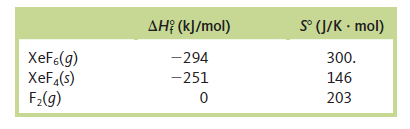Chemistry Practice Problems Entropy Practice Problems Solution: Given the thermodynamic data below, calculate ΔS a...

⚠️Our tutors found the solution shown to be helpful for the problem you're searching for. We don't have the exact solution yet.

# Solution: Given the thermodynamic data below, calculate ΔS and ΔS surr for the following reaction at 25°C and 1 atm:                       XeF  6(g) →XeF4(s) + F2(g)

###### Problem

Given the thermodynamic data below, calculate ΔS and ΔS surr for the following reaction at 25°C and 1 atm:

XeF  6(g) →XeF4(s) + F2(g)Entropy

Entropy

#### Q. Without doing any calculations, determine the sign of ΔSsys for each of the following chemical reactions.a. C3H8(g) + 5 O2(g) → 3 CO2(g) + 4 H2O(g), Δ...

Solved • Thu Jan 03 2019 09:30:35 GMT-0500 (EST)

Entropy

#### Q. Consider these three changes in the possible distributions of six gaseous particles within three interconnected boxes. Which change has a positive ΔS?

Solved • Thu Jan 03 2019 09:30:32 GMT-0500 (EST)

Entropy

#### Q. For rubidium ΔH°vap = 69.0 kJ/mol at 686°C, its boiling point. Calculate ΔS°, q, w, and ΔE for the vaporization of 1.00 mole of rubidium at 686°C and ...

Solved • Mon Dec 03 2018 16:41:27 GMT-0500 (EST)

Entropy

#### Q. Rank the contents of the following containers in order of decreasing entropy.

Solved • Mon Dec 03 2018 16:17:04 GMT-0500 (EST)